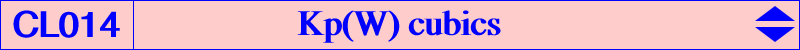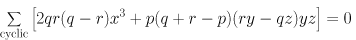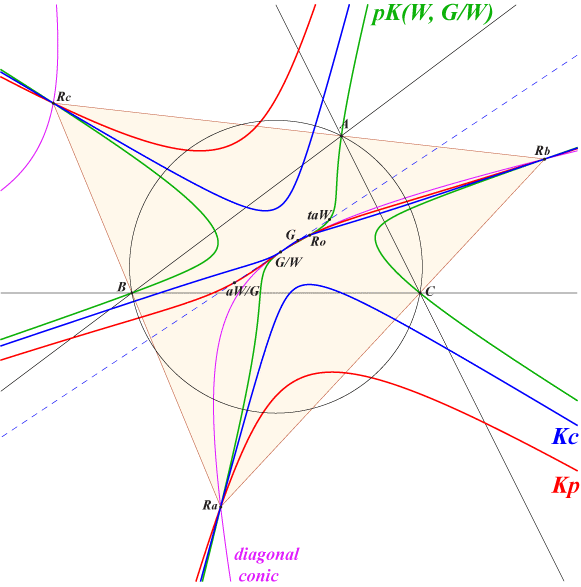Let W = (p : q : r) be a point. Kp(W) is the locus of pivots of all pK+ invariant under the isoconjugation with pole W. Kc(W) is the locus of the common point of the three asymptotes. Compare with the classes CL017 and CL018. If W = G, these two cubics Kp(W) and Kc(W) degenerate into the medians of ABC. This is excluded in the sequel. Kp(W) and Kc(W) are always K+ cubics and, for a given W, they have the same asymptotic directions. The asymptotes of Kp(W) concur at the reflection Xp of G about G/W and those of Kc(W) at the homothetic Xc of G/W under h(G,2/3). Kc(W) is actually the homothetic image of Kp(W) under h(G/W, -1/3) where G/W = G-Ceva conjugate of W, the center of the circum-conic with perspector W. Both cubics contain the (real or not) square roots Ro, Ra, Rb, Rc of W and G/W with a common tangent passing through G. Hence, Kp(W) and Kc(W) generate a pencil of cubics containing pK(W, G/W) and the degenerate cubic into the line at infinity and the diagonal conic passing through W and the square roots of W. The tangential of G/W in Kc(W) is G and the tangential of G/W in Kp(W) is aW/G, the homothetic of G/W under h(G,4). The tangents to Kp(W) at the four fixed points of the isoconjugation concur at W. This is false for Kc(W). Kp(W) and Kc(W) are K60+ cubics if and only if W = X(6). See Kp60 = K077 and Kc60 = K078. Kp(W) and Kc(W) are K++ cubics if and only if W lies on the Steiner inscribed ellipse but Kc(W)++ always degenerates since G/W lies on the line at infinity and coincide with Ro. The cubics Kp(W)++ form the class CL016 of cubics. Kp(W) contains the following points : the (real or not) fixed points Ro, Ra, Rb, Rc of the isoconjugation i.e. square roots of W giving decomposed cubics pK. their homothetic images under h(G/W, -3) the centre of the conic isoconjugate of the line at infiny which is G/W. In this case, the asymptotes of pK(W, G/W) concur at G. W/G = W-Ceva conjugate of G. aW/G where aW is the anticomplement of W. In this case, the asymptotes of pK(W, aW/G) concur at G/W. the infinite points of pK(W, G/W). The barycentric equation of Kp(W) is :Kp(W) is a pivotal cubicThe polar conic of aW/G meets Kp(W) at aW/G (double), G/W and three other points A1, B1, C1. The polar conic of W/G meets Kp(W) at W/G (double), W/G and three other points A', B', C'. The diagonal triangle of the quadrilateral A1, B1, C1, G/W is A'B'C'. Kp(W) is the pivotal cubic with pivot aW/G and isopivot W/G with respect to this triangle A'B'C'. Note that A1, B1, C1, G/W are the fixed points of the isoconjugation hence the tangents to Kp(W) at these points pass through the pivot aW/G. Similarly, since A', B', C' are the singular points of the isoconjugation, the tangents at these points and at the pivot aW/G pass through the isopivot W/G. Recall that the tangents at Ro, Ra, Rb, Rc pass through W.